Courses
Courses for Kids
Free study material
Free LIVE classes
More

# Can You See The Pattern Class 5 Notes CBSE Maths Chapter 7 (Free PDF Download)LIVE
Join Vedantu’s FREE Mastercalss

## NCERT Class 5 Maths Chapter 7 Revision Notes and Worksheets: Simple Illustration of Patterns

Vedantu offers free PDF for NCERT Class 5 Maths Chapter 7 revision notes and worksheets for students’ practice purposes. You must have studied patterns and learned how to recognize them in the previous classes. In Class 5, you will proceed to the next level of recognizing patterns and how to solve the problems on patterns. This chapter will help you develop your skills to draw patterns after rotating them. Download and refer to the Can You See The Pattern revision notes from Vedantu to understand the advanced concepts easily.

The revision notes come with worksheets that you can solve for practicing these problems. You will learn how to recognize patterns and use your logical reasoning to find the sequences.

Last updated date: 19th Sep 2023
Total views: 144k
Views today: 4.43k

## Patterns

• A pattern is referred to as the arrangement of shapes, numbers, and colours which are in repetition.

• Number patterns, image patterns, logic patterns, word patterns, and so on are all examples of patterns in mathematics.

## Image Patterns / Shapes Pattern

• Image Pattern is referred to as the sequence followed by a certain group of objects/shapes.

• For example, In the below image, we can see that the umbrellas follow a pattern. The umbrella is pointing down, then left, then up, and then back to the right. As a result, the following pattern can be observed: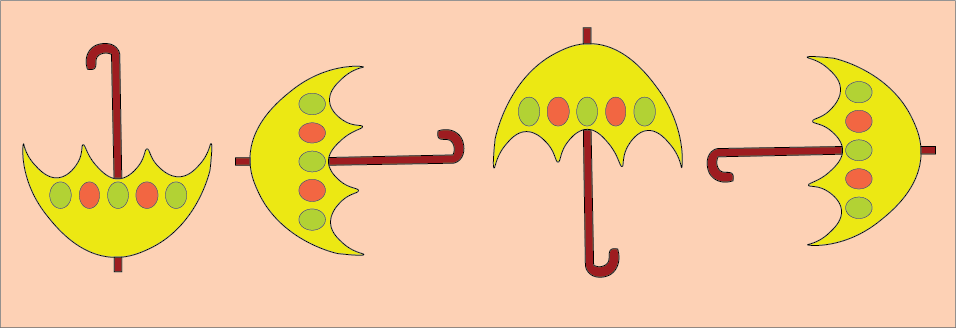Shape pattern

## Number Patterns

• A list of numbers follows a specific sequence based on a rule. Such as algebraic or arithmetic, geometric, and the Fibonacci pattern.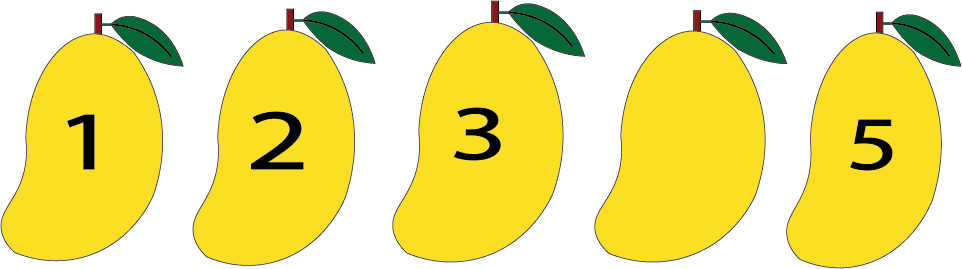Shape pattern of mangoes

## Arithmetic or Algebraic Patterns

• Using addition or subtraction we can find the missing number in a sequence.

• The difference between the first and second numbers in the below figure is +3.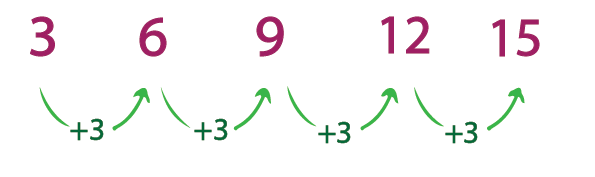Arithmetic Progression

Solved Example 1: Complete the following number patterns?

5, 10,15 , __, __, __

Ans. First Digit = 5

Second Digit = 5 + 5 = 10

Third Digit = 10 + 5 = 15

Clearly, we will add 5 to the preceding digits respectively to find the rest of the digits.

Fourth Digit = 15 + 5 = 20

Similarly , Fifth Digit = 20 + 5 = 25

Sixth Digit = 25 + 5 = 30

Hence the pattern is 5,10,15,20,25,30.

## Geometric Series Pattern

• A geometric pattern is a series of numbers formed by multiplying and dividing them.

• Example: Here multiply each term by 2 to get the next term.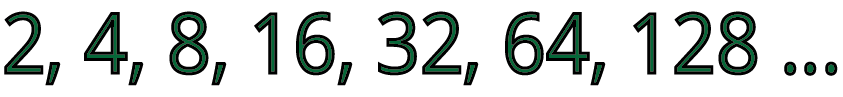Geometric series

Solved Example 1: Complete the following number patterns?

10, 100,1000 , __, __, __

Ans. First Digit = 10

Second Digit = 10 × 10 = 100

Third Digit = 100  × 10 = 1000

Clearly, we will multiply 10 by the preceding digits respectively to find the rest of the digits.

Fourth Digit = 1000  × 10 = 10000

Similarly , Fifth Digit = 10000  × 10 = 100000

Sixth Digit = 100000  × 10 = 1000000

Hence the pattern is 10,100,1000,10000,100000,1000000.

## Fibonacci Pattern

• The Fibonacci Pattern is a number sequence in which each consecutive number is obtained by adding the two preceding numbers together.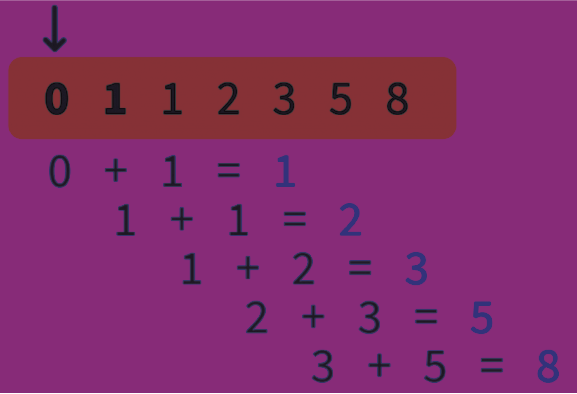Fibonacci Pattern

## Equal Numbers

• When two or more quantities are exactly the same, we can say they are equal.

• In number patterns, if there is an equal sign(=) in the pattern, then LHS and RHS will have the same numbers.

Solved Examples 1: Find the missing numbers in the following pattern: 10, 20, _ = 10, _, 15.

Ans: As you can see, there are two numbers in the LHS: 10 and 20.

And in RHS: 10 and 15

As there is an equal sign it means both sides will have the same numbers.

So the missing numbers in the LHS = 15

And, the missing number in the RHS = 20

Numbers in LHS: 10, 20, 15

Numbers in RHS: 10, 20, 15

Numbers in LHS = Numbers in RHS

Therefore LHS = RHS

Solved Examples 2. Check whether LHS is equal to RHS: 15+ _ + _ = 30 + 20 + 15 ?

Ans: As you can see, there are three numbers in the RHS: 30 + 20 + 15.

And in LHS only 15

As there is an equal sign it means both sides will have the same numbers.

So the missing numbers will be the numbers that are in the RHS i.e, 30 and 20

So, missing numbers in LHS will be 15 + 30  + 20

If you add both LHS and RHS we get,

LHS: 15 + 30  + 20 = 65

RHS: 30 + 20 + 15 = 65

Therefore LHS = RHS

## Palindrome

• Special words/numbers which remain the same when reversed.

• For example, 99 when reversed remains 99; 101, when reversed, remains 101.

## Fun with Odd Numbers

• Integers that do not appear in the table of two are known as odd numbers.

• Note: When we add the first n odd numbers, we will get the sum as n × n, where (n) is the number of odd numbers taken at a time.

Solved Example 1: Let us find the sum of the first 3 odd numbers.

Ans: First 3 odd numbers: 1,3, 5. Here n = 3

Also, we know that When we add first n odd numbers,

we will get the sum as n × n.

Sum of first 3 odd numbers = n × n i.e. 3 × 3 = 9

So, the Sum of the first 3 odd numbers is 9.

Solved Example 2: Find the sum of the first 6 odd numbers.

Ans: First 6 odd numbers: 1,3, 5,7,9,11. Here n = 6

Also, we know that when we add first n odd numbers,

we will get the sum as n × n.

Sum of first 6 odd numbers = n × n i.e. 6 × 6 = 36

So, the Sum of the first 6 odd numbers is 36.

## Practice Questions:

Q1. Complete the following number patterns?

• 20, 30, 40 , __, __, __

• 3, 5, 7 , __, __, __

Ans:

• 50, 60, 70

• 9, 11, 13

Q2. Find the sum of the first 11 odd numbers.

Ans.

• 121

Q3. Find the missing numbers in the following pattern: 10, 20, _, 40, 50 = 10, _, 30,_,_.

Ans.

• Missing numbers in LHS = 30

• Missing numbers in RHS = 20,40 and 50.

## Importance of CBSE Class 5 Maths Chapter 7 Can You See the Pattern

• This chapter will progress to the next level where you can identify the patterns and follow the changes in the designs.

• You will learn how to rotate an image or a shape to find the next shape or phase of a sequence. These types of problems related to patterns do not follow any particular mathematical concept. In fact, you will not need any mathematical operations to do. All you will learn is to identify the patterns, check the sequential flow of these patterns, and solve the questions.

• This chapter promotes logical thinking among students. If you follow the Can You See The Pattern summary, you will clearly understand the objective of this chapter.

• You will learn at what angle the patterns, designs, images, letters, numbers, etc., need to be rotated to find the answers to such questions.

## Benefits of Can You See The Pattern Class 5 Worksheets and Revision Notes

• Our subject experts have come up with a concise summary of all the fundamental concepts taught in this chapter. It will help you to grab these concepts easily and accurately solve the questions.

• This chapter enhances the development of your aptitude. Your logical reasoning skills to look for a pattern will increase considerably once you start practising this chapter. To support this development, you will need to practice the worksheets and revision notes.

• Learn how the number patterns are changing by checking the solutions and explanations provided by our subject experts.

• Prepare for your exam by using the revision notes to revise the concepts you have studied in this chapter, quickly.

## Easy Exam Preparation with Class 5 Maths Chapter 7 Worksheets and Revision Notes

Download these revision notes and worksheets for free from Vedantu and learn how the experts have solved magic squares and hexagons problems. Follow the steps used by the experts and solve the worksheets to find out how good you are at solving these questions. Practice the sums thoroughly to score well in the exams.

## FAQs on Can You See The Pattern Class 5 Notes CBSE Maths Chapter 7 (Free PDF Download)

1.  How to learn and revise Class 5 Maths Can You See The Pattern?

Your first step is to follow the classroom sessions. Proceed to learn the concepts covered in this chapter and solve the exercise problems. Download and refer to the NCERT Solutions for Class 5 Chapter 7 and find out how the experts have solved them. Move on to the worksheets provided with the revision notes. Learn where you need to improve yourself to solve the questions. Once done, use the revision notes to revise the concepts before your exam.

2.  Will I be able to learn the rules to make patterns?

Yes. Follow how the experts have explained this rule in the revision notes. Relate the explanations given with that of the textbook. It will help you find out the patterns easily and answer the questions.

3.  How can I find the patterns in number hexagons?

Check the shape of the boxes and their positions. You will find three numbers on each side. Use mathematical operations to find their relations. This is how you can find a pattern.